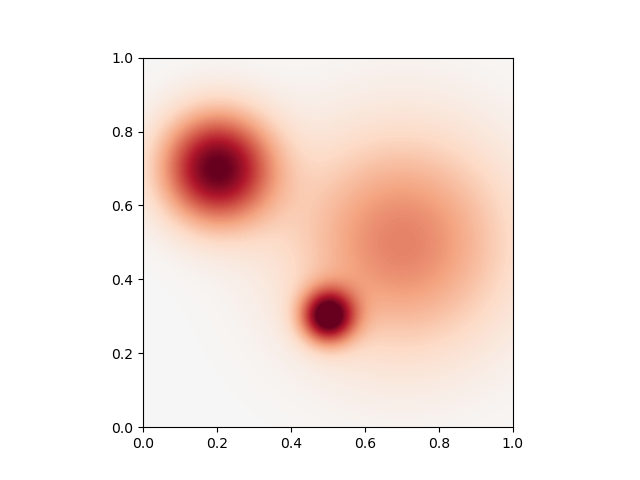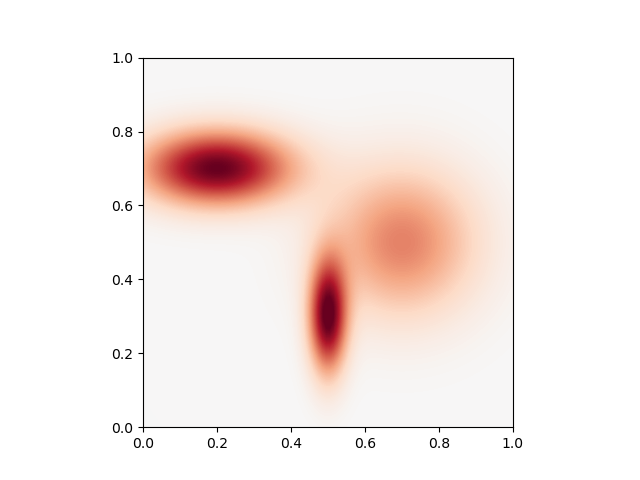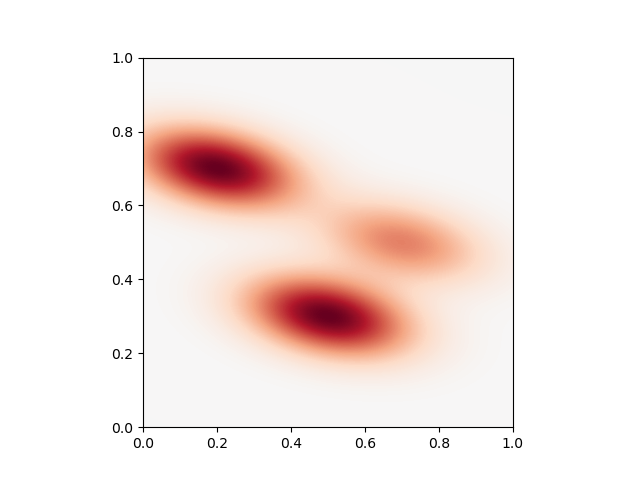# Anisotropic kernels¶

Let’s see how to encode anisotropic kernels with a minimal amount of effort.

## Setup¶

Standard imports:

import numpy as np
from matplotlib import pyplot as plt
import matplotlib.cm as cm
import torch
from pykeops.torch  import Kernel, kernel_product


Dataset:

# Choose the storage place for our data : CPU (host) or GPU (device) memory.
dtype = torch.cuda.FloatTensor if torch.cuda.is_available() else torch.FloatTensor

# Three points in the plane R^2
y = torch.tensor( [
[ .2, .7],
[ .5, .3],
[ .7, .5]
]).type(dtype)

# Three scalar weights
b = torch.tensor([
1., 1., .5
]).type(dtype)

# Remember that KeOps is super-picky on the input shapes:
# b is not a vector, but a 'list of unidimensional vectors'!
b = b.view(-1,1)

# Create a uniform grid on the unit square:
res = 100
ticks  = np.linspace( 0, 1, res+1)[:-1] + .5 / res
X,Y    = np.meshgrid( ticks, ticks )

# Beware! By default, numpy uses float64 precision whereas pytorch uses float32.
# If you don't convert explicitely your data to compatible dtypes,
# PyTorch or Keops will throw an error.
x = torch.from_numpy(np.vstack( (X.ravel(), Y.ravel()) ).T).contiguous().type(dtype)

def plot_kernel(params) :
""" Samples 'x -> ∑_j b_j * k_j(x - y_j)' on the grid, and displays it as a heatmap. """
heatmap   = kernel_product(params, x, y, b)
heatmap   = heatmap.view(res,res).cpu().numpy() # reshape as a 'background' image
plt.imshow(-heatmap, interpolation='bilinear', origin='lower',
vmin = -1, vmax = 1, cmap=cm.RdBu,
extent=(0,1,0,1))
plt.show()


## Kernel definition¶

Let’s use a Gaussian kernel given through

$k(x_i,y_j) = \exp( -\|x - y\|_{\Gamma}^2) = \exp( - (x_i - y_j)^t \Gamma (x_i-y_j) ),$

which is equivalent to the KeOps formula exp(-WeightedSquareNorm(gamma, x_i-y_j )). Using the high-level pykeops.torch.Kernel and pykeops.torch.kernel_product() wrappers, we can simply define:

params = {'id' : Kernel('gaussian(x,y)')}


The precise meaning of the computation is then defined through the extra entry gamma of the params dictionary, which will is to be used as a ‘metric multiplier’. Denoting D == x.shape == y.shape the size of the feature space, the integer K can be 1, D or D*D. Rules are:

• if gamma is a vector (gamma.shape = [K]), it is seen as a fixed parameter

• if gamma is a 2d-tensor (gamma.shape = [M,K]), it is seen as a j-variable

N.B.: Beware of Shape([K]) != Shape([1,K]) confusions!

## Isotropic Kernels¶

If K == 1 (ie gamma is a float): $$\Gamma = \gamma Id_D$$ is a scalar factor in front of a simple Euclidean squared norm. In this case, WeightedSquareNorm(gamma, x-y ) corresponds to

$\|x - y\|_{\Gamma}^2 = \gamma \|x-y\|^2$

### Uniform kernels¶

Providing a single scalar we get uniform kernels

sigma = torch.tensor( [0.1] ).type(dtype)
params['gamma'] = 1./sigma**2
plt.plot()
plot_kernel(params)### Variable kernels¶

Providing a list of scalar we get variable kernels

sigma = torch.tensor( [
[0.15],
[0.07],
[0.3]
]).type(dtype)
params['gamma'] = 1./sigma**2
plot_kernel(params)## Diagonal Kernels¶

If K == D (ie gamma is a vector): $$\Gamma = \text{diag}(\gamma)$$ is a diagonal matrix. In that case, WeightedSquareNorm(gamma, x-y) corresponds to

$\|x - y\|_{\Gamma}^2 = \langle (x-y), \Gamma (x-y) \rangle = \langle (x-y), \text{diag}(\gamma) (x-y) \rangle = \sum_d \gamma_d (x_d-y_d)^2$

### Uniform kernels¶

Providing a single vector we get uniform kernels

sigma = torch.tensor( [0.2, 0.1] ).type(dtype)
params['gamma'] = 1./sigma**2
plot_kernel(params)### Variable kernels¶

Providing a list of vector (ie a 2d-tensor) we get variable kernels

sigma = torch.tensor( [
[0.2, 0.1],
[.05, .15],
[.2,  .2]
] ).type(dtype)
params['gamma'] = 1./sigma**2
plot_kernel(params)## Fully-Anisotropic kernels¶

If K == D*D (ie gamma is a vector of size the dimension of the ambiant space squared): $$\Gamma$$ is a symmetric matrix whose entries are stored in $$\gamma$$. In that case, WeightedSquareNorm(gamma, x-y) corresponds to

$\|x - y\|_{\Gamma}^2 = \langle (x-y), \Gamma (x-y) \rangle = \sum_{k}\sum_{\ell} g_{k,\ell} (x_k-y_k)(x_\ell-y_\ell) )$

### Uniform kernels¶

Providing a single vector we get uniform kernels

Sigma = torch.tensor( [1/0.2**2, 1/.25**2, 1/.25**2, 1/0.1**2 ] ).type(dtype)
params['gamma'] = Sigma
plot_kernel(params)### Variable kernels¶

Providing a list of vector (ie a 2d-tensor) we get variable kernels

Sigma = torch.tensor( [
[1/0.2**2, 1/.25**2, 1/.25**2, 1/0.1**2  ] ,
[1/0.1**2,     0,       0,     1/0.12**2 ] ,
[1/0.3**2,-1/.25**2,-1/.25**2, 1/0.12**2 ] ,
] ).type(dtype)

params['gamma'] = Sigma
# sphinx_gallery_thumbnail_number = 6
plot_kernel(params)Total running time of the script: ( 0 minutes 0.472 seconds)

Gallery generated by Sphinx-Gallery